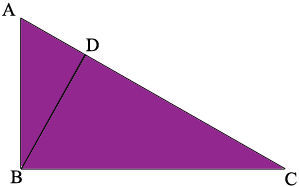SEARCH HOMEMath Central Quandaries & QueriesQuestion from LYNDELL: I have a right triangle and know the length of all sides. How do I calculate the shortest distance from the vertex of the 90 degree angle to the hypotenuse?Hi Lyndell,

In the right triangle below $D$ is the point on $CA$ which is closest to $B.$The angle $CDB$ is a right angle. Can you see why? What do you know about the relationship between the triangles $CBD$ and $CAB?$

PennyMath Central is supported by the University of Regina and The Pacific Institute for the Mathematical Sciences.